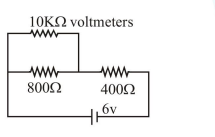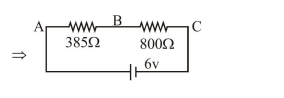# Two resistors of resistance 400Ω and 800Ω

Question:

Two resistors $400 \Omega$ and $800 \Omega$ are connected in series across a $6 \mathrm{~V}$ battery. The potential difference measured by a voltmeter of $10 \mathrm{k} \Omega$ across $400 \Omega$ resistor is close to:

1. $2 \mathrm{~V}$

2. $1.95 \mathrm{~V}$

3. $2.05 \mathrm{~V}$

4. $1.8 \mathrm{~V}$

Correct Option: , 2

Solution:So the potential difference in voltmeter across

the points $\mathrm{A}$ and $\mathrm{B}$ is $\frac{6}{1185} \times 385=1.949 \mathrm{~V}$